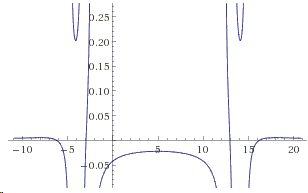The following problem has been offered at the 2006 Singapore Secondary Schools Mathematical Olympiad for Junior Section [Lecture Notes, p 3]:

Suppose that the two roots of the equation

$\displaystyle \frac{1}{x^{2}-10x-29}+\frac{1}{x^{2}-10x-45}-\frac{2}{x^{2}-10x-69}=0$

are $\alpha$ and $\beta$. Find the value of $\alpha +\beta$.

On my attempt to solve the problem I have copied it this way:

$\displaystyle \frac{1}{x^{2}-10x-29}+\frac{1}{x^{2}-10x-45}-\frac{1}{x^{2}-10x-69}=0.$

Having figured out a solution I was surprised to discover that my equation had four roots - instead of two. However, the answers were not unrelated. I soon discovered my error and realized where the relation between the two answers came from. Both equations belong to a certain kind; the kind that allows one to see the sum of its roots practically at a glance.

Solution

### References

1. Xu Jiagu, Lecture Notes on Mathematical Olympiad Courses, v 8, (For senior section, v 1), World Scientific, 2012Suppose that the two roots of the equation

$\displaystyle \frac{1}{x^{2}-10x-29}+\frac{1}{x^{2}-10x-45}-\frac{2}{x^{2}-10x-69}=0$

are $\alpha$ and $\beta$. Find the value of $\alpha +\beta$.

It is hard to miss the fact that the three denominators all contain $x^{2}-10x$. After completing the square, introduce a new variable:

$y=(x-5)^2.$

In terms of the so defined $y$ the equation becomes:

$\displaystyle \frac{1}{y-54}+\frac{1}{y-70}=\frac{2}{y-94}$

which can be rewritten as

$(2y-124)(y-94) = 2(y-54)(y-70),$

or else

$2y^{2}-312y + 124\cdot 94 = 2y^2 - 248y + 2\cdot 54\cdot 70,$

which is a linear equation $64y = 4096$. From which $y =64$. This leads to an equation in $x$:

$(x-5)^2 = 64,$

so that, say, $\alpha = 13$ and $\beta =-3$, making $\alpha+\beta=10$.

The same substitution in the mistyped case leads to a quadratic equation in $y$, i.e., a quartic equation in $x$. As a matter of fact, in order to find the sum of the roots of the polynomial equation of degree $n,$ one only has to calculate the coefficient by $x^{n-1}$ (Viète's theorem). But having to first solve an equation in $y$, we then split the problem into solving several (1 or 2 or, in principle, more) quadratic equations, the sum of whose roots in the two cases at hand is $10$. Thus the answer in my (mistyped) case was "the sum of the four roots is 20".

Below are two graphs (of the left-hand sides), one with two roots, the other with four:Where have the numbers $10$ and $20$ come from? Clearly, the source is the $10$ in the common part of the denominators $x^2-10x \ldots$ And next comes the insight:

The sum of all roots of an equation $f(x)=0$, where $f$ is an even function, is $0$.

Now we adapt that to the equation at hand:

The sum of all roots of an equation $f(x-a)=0$, where $f$ is an even function, is a multiple of $2a$. More accurately, the sum of the roots is equal to $\displaystyle 2a\cdot\frac{n}{2}$, where $n$ is the number (necessarily even) of roots.

With this in mind, you can modify the free terms in the denominators or the numerators and still get $10$ or $20$ as the sum of all roots; the answer being dependent on the number of roots or, in other words, on whether the simplified equation comes out linear or quadratic. This can be determined at a glance. Say, the sum of the roots of the equation below is $-8$, even if some of the roots may be complex.

$\displaystyle \frac{2}{x^{2}+8x-29}+\frac{5}{x^{2}+8x-45}-\frac{7}{x^{2}+8x-69}=0.$

In a more general case, you do not even need to solve an equation in $y$, but only ascertain its degree - the equation in $x$ will have twice as many roots.